# The Deutsch-Joza Algorithm

### Contents

1. The Problem
2. Classical Solution
3. The Algorithm

### The Problem

#### Black Boxes

You are probably (hopefully) familiar with the style of computation in which our problem is coded into our input bits and we perform operations on these bits until our output bits contain our answer. For example, an addition circuit may take two binary numbers in two registers (we call a set of bits a register) and output their sum into one of these registers: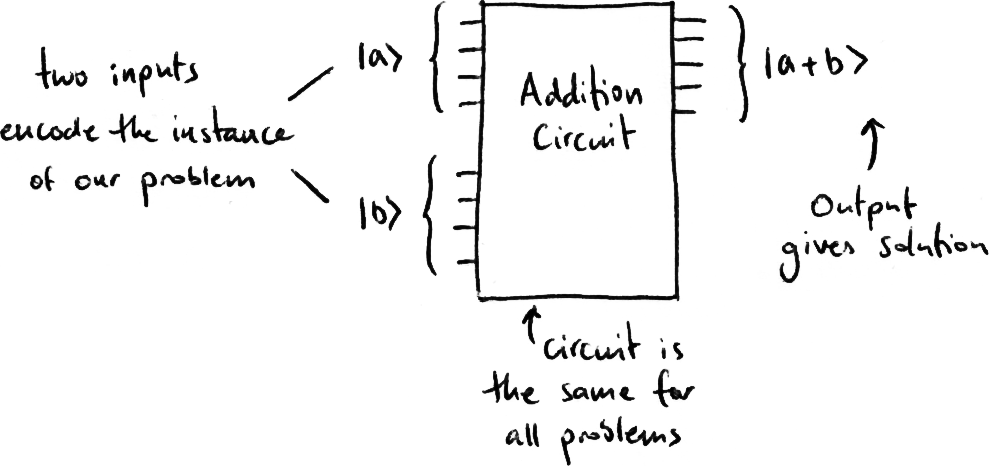Here is an example of this provided by the creator of Quirk:

In quantum computing we often use a different style of computation, solving what we call a ‘black box’ problem. A black box is simply a quantum circuit that we are not allowed to look at or take apart. In this case, the instance of the problem is encoded into the operations we perform instead of the input registers: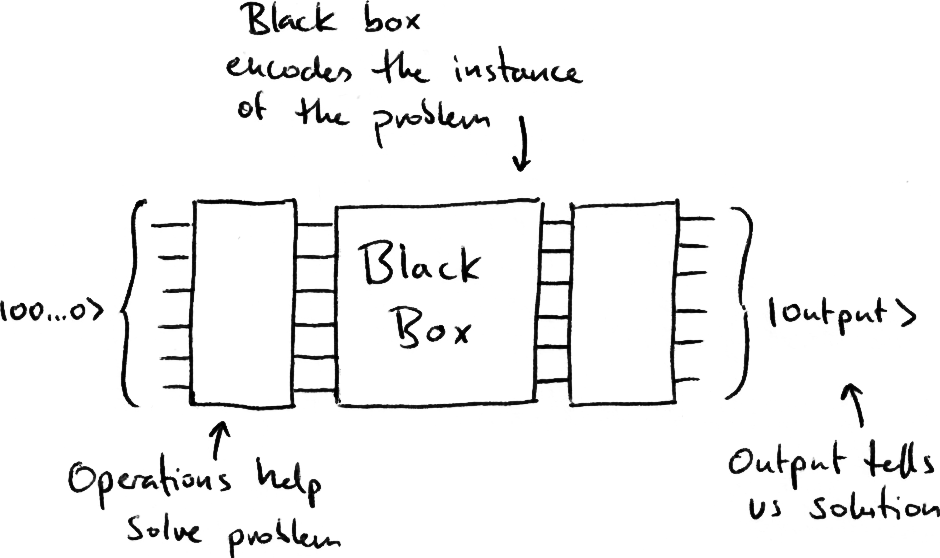It might seem difficult to understand how a black box problem can be useful, but we will address this in other articles (such as in the Grover's Algorithm section).

#### The Deutsch-Joza Black Box Problem

We are given a classical black box that takes n input qubits, and outputs to one output qubit: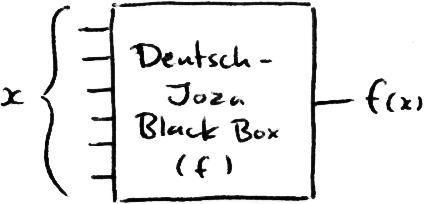To make the circuit reversible we need to adapt our black box so it has the same number of input qubits as output qubits. In our case we give the circuit n input qubits plus one extra qubit for output, the circuit returns the original n input qubits in their initial state, plus the output qubit in its new state.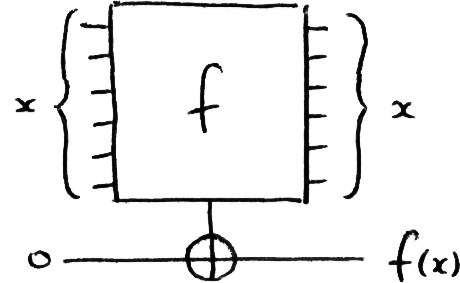We are guaranteed that the output of this black box is either constant or balanced:

• Constant: The state of the output bit is the same for all input states
• Balanced: The output bit is ON for half of the input states and OFF for the other half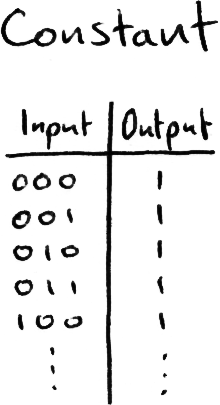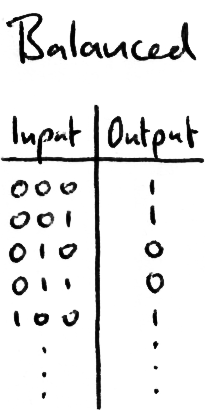The problem is to find out whether the black box is constant or balanced.

### The Classical Solution

Classically, we can solve this problem by passing the box different input states and recording the output. If the box is balanced we will need at least two queries in the best case: one output ON and one output OFF. On average we will need more than this.

To prove the box is constant with 100% certainty requires us checking one more than half of the possible input states (2n-1 + 1 queries). Though if we are willing to accept a small margin of error we need a lot less queries than this. We can see that the classical solution needs between 2 queries (in the best case) to 2n-1 +1 queries (in the worst case). The Deutsch-Joza algorithm only ever needs one query.

### The Algorithm

The Deutsch-Joza algorithm is simple:

1. Set the output qubit to |-⟩
2. Apply H-gates to the n input qubits
3. Run the black box
4. Apply H-gates to the n input qubits
5. Measure the n input qubits:
• If all n qubits are in the state |0⟩, the box is constant
• Otherwise, the box is balanced

We can draw this as a quantum circuit: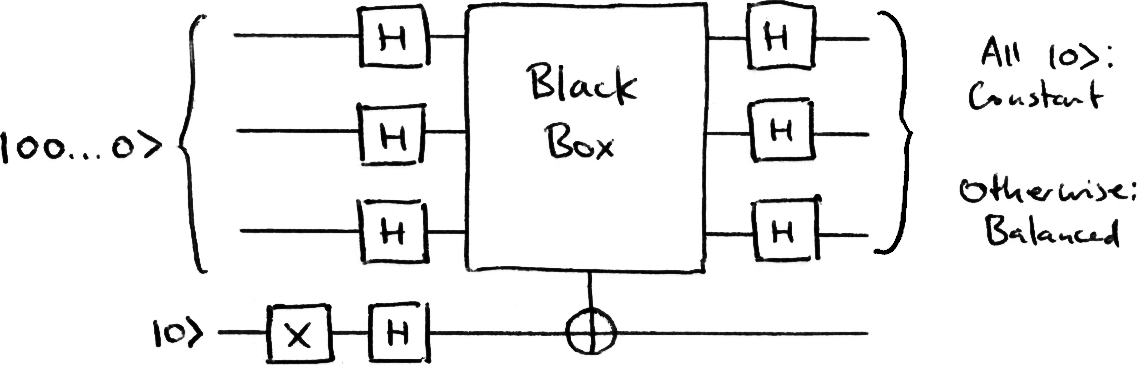To keep with the labelling of the reversible classical circuit above, I will still refer to the top n qubits as the ‘input’ qubits, and the bottom qubit as the ‘output’ qubit. Bear in mind that after the black box we ignore the bottommost qubit, so the top n qubits could be considered the output of the algorithm. I have provided a link (below) to an example in Quirk. This example has six custom gates (located in the lower right corner), each takes the first qubits as input and the final qubit as output. Use the Deutsch-Joza algorithm in Quirk to find out if each black box is constant or balanced: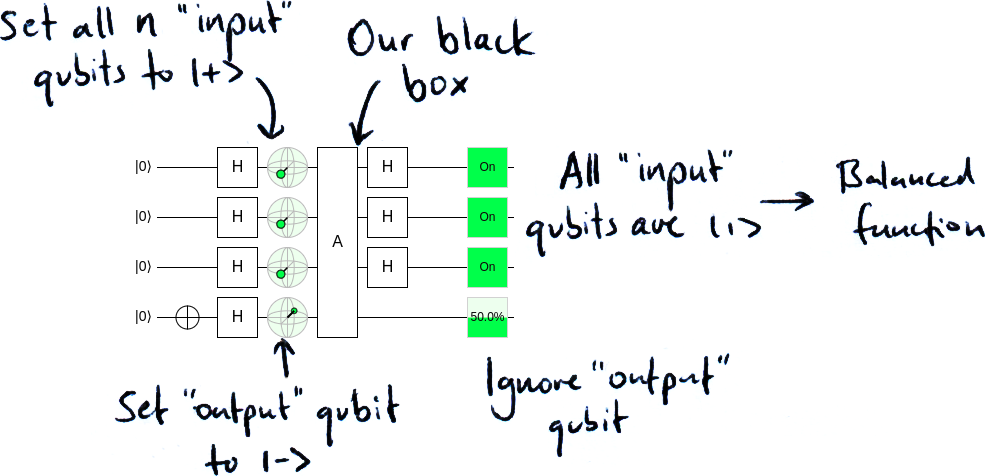You should also try creating your own black box circuits and see how the algorithm behaves when given neither a balanced nor constant circuit.